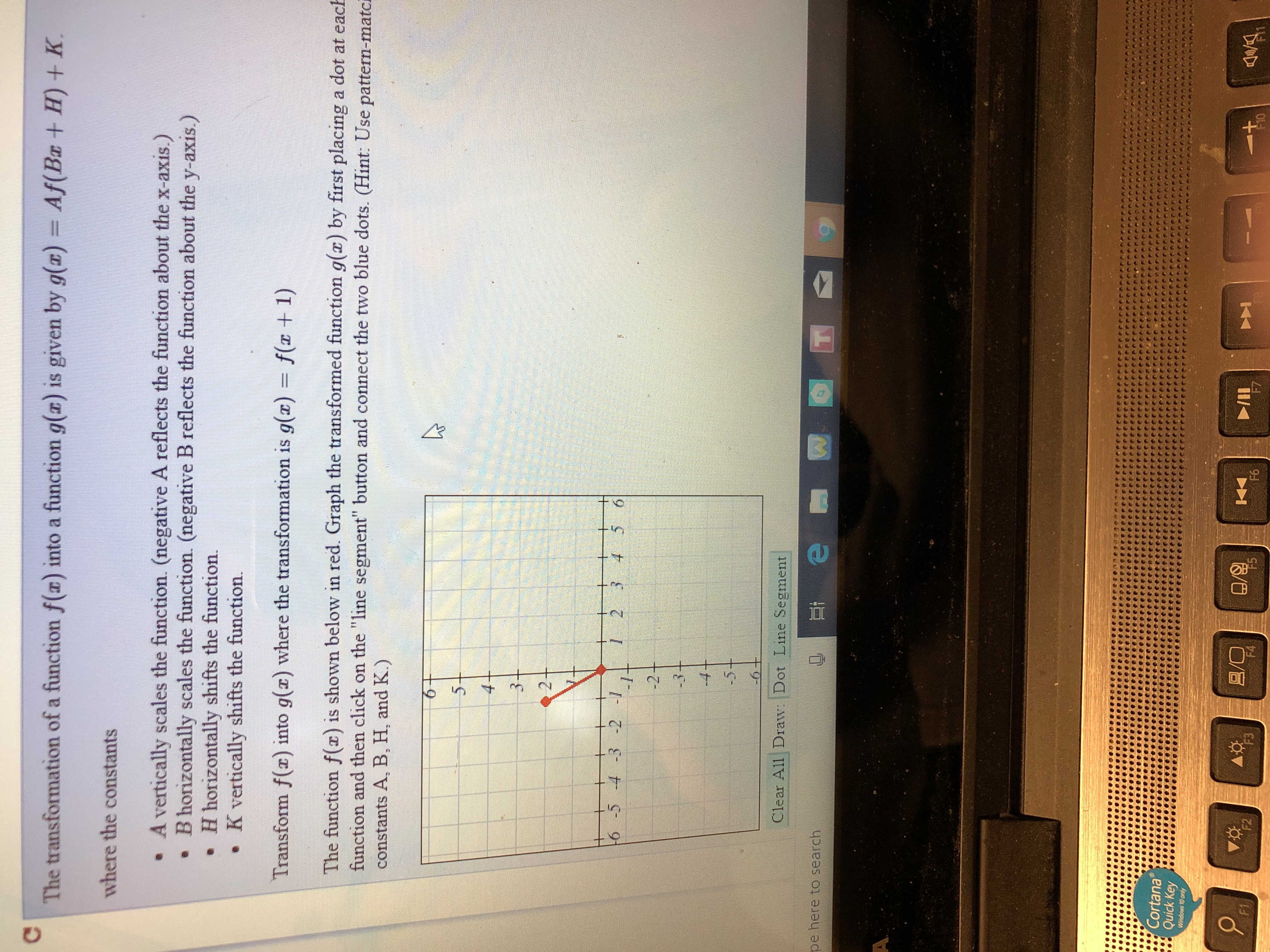# The transformation of a function f(x) into a function g(x) is given by g(a) Af(BaH)Kwhere the constantsA vertically scales the function. (negative A reflects the function about the x-axis.)B horizontally scales the function. (negative B reflects the function about the y-axis.)H horizontally shifts the function.. K vertically shifts the function.Transform f() into g() where the transformation is g(z) f 1)The function f(x) is shown below in red. Graph the transformed function g(z) by first placing a dot at eacfunction and then click on the "line segment" button and connect the two blue dots. (Hint: Use pattern-matcconstants A, B, H, and K.)5-26 -5 4 3 2 12 3 4 5 6Clear All Draw·Dot LineSegmentpe here to searchCortanaQuick KeyWindows 10 onlyPMF2ovgF4F610

Question
122 viewshelp_outlineImage TranscriptioncloseThe transformation of a function f(x) into a function g(x) is given by g(a) Af(BaH) K where the constants A vertically scales the function. (negative A reflects the function about the x-axis.) B horizontally scales the function. (negative B reflects the function about the y-axis.) H horizontally shifts the function. . K vertically shifts the function. Transform f() into g() where the transformation is g(z) f 1) The function f(x) is shown below in red. Graph the transformed function g(z) by first placing a dot at eac function and then click on the "line segment" button and connect the two blue dots. (Hint: Use pattern-matc constants A, B, H, and K.) 5- 2 6 -5 4 3 2 12 3 4 5 6 Clear All Draw·Dot LineSegment pe here to search Cortana Quick Key Windows 10 only PM F2 ovg F4 F6 10 fullscreen
check_circle

Step 1

Given graph is:

Step 2

In the transformation of the function g (x) = Af (Bx + H) + K

A scales the function vertically, B scales the function horizontally, H shifts the function horizontally and K shifts the function vertically.

In the given transformation g (x) = f (x + 1),

A = 1, B = 1, H = 1 and K = 0.

Since A and B represent scaling and the scaling factor here is 1, the graph is not scaled.

Since K is 0, the graph does not shift vertically.

But as H is 1, the graph shifts one unit to the left.

Step 3

The points on the given graph of f (x) are (0,0) and (-1,2)

So after transformation, the points are (-1,0) and (-2,2)

Join...

### Want to see the full answer?

See Solution

#### Want to see this answer and more?

Solutions are written by subject experts who are available 24/7. Questions are typically answered within 1 hour.*

See Solution
*Response times may vary by subject and question.
Tagged in

### Other